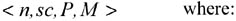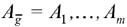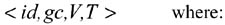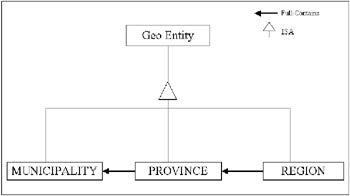# BASIC NOTIONS

In this section, we describe some basic notions of multidimensional data and geographic data needed to define the cooperation approach. They are based on the common concepts discussed extensively in several proposals.

### Geographic Data

The geographical data model under consideration is based on the existence of sets and definitions discussed by Pourabbas & Rafanelli (2000) as follow:

• a finite set of domains D = {D1,, Dn}

• an infinite numerical set A = {A1,, An} of symbols called instance variables

• an infinite numerical set of identifiers

• set C of all the classes defined in the database

• set O of all the objects defined in the database

• set M of all the methods defined in the database.

A geographical class is a set of elements called geographical objects go1, go2,, gon, which have the same properties A1, A2,, An, defined over the set of not necessarily distinct domains D1, D2,, Dn, and a set of methods which describe the behavior of the objects.

Definition 3: a geographic class (gc) is a quadruple:• n is the name of gc;

• sc is the class-parent of gc;

• P is the set of attributes which define the geographic entity properties enclosing the set of attributes inherited from the relative super-class sc. It consists of:

• a geometric attribute Ag, which is the type of geometric structure that refers to the geographic class. It can assume only one value chosen in its domain definition: {null, point, polyline, region};

• a set of alphanumeric attributesdefined on domains D1,, Dm which are the non-geometric properties of the gc instances; each of them consists of a couple (attribute name, data type);

• M is the non empty set of methods such that: M ={inherited methods from sc } {methods defined on gc} {overridden methods}

Example 1: The geographic class "province" is defined as: <province, administrative subdivision, {{(name, string), (surface, integer), (population, integer),..}, region }, {Get_name, }>

Definition 4: A geographic object go instance of a geographic class gc represents a geographic entity of the real world. It consists of a quadruple:• id is the identifier of a geographic class instance; it is unique.

• gc is the class to which it belongs; it is also unique.

• V represents the ordered set of the alphanumeric attribute values.

• T is the ordered set of couples (latitude, longitude) which specifies the object position with regard to the geographic coordinates.

Example 2: Let us consider an instance of the geographic class "province": <001, province, {(name, Roma), (surface, 1507.60 km2), (population, 2915000),.}.{(x1,y1),, (xn,yn)}>

Definition 5: The root class (rt) is the superclass of all the geographic classes. It is a quadruple <n,sc,P,M>, where sc = Φ (void class) because its parent does not exist.

If the geometric attribute value is null, the geographic class is the null geographic class and the quadruple is defined as <n, rt, P, Φ>, where n = "void class," P = < Φ, null>, where Φ represents the set of alphanumeric attributes which defines the non-geometric characteristics of the gc instances, and null represents the type of geometric structure of the instances.

Definition 6: A method is a triple

<nm, f : U R, s >, where:

• nm is the name of the method.

• f : U R is the method's interface in the form of: f : U1 × U2 ×× Un R where U1, U2, Un are the domains of the arguments, R is the domain of the result, and U1 = ist(gc), where ist(gc) represents the class instances.

• s is the set of instructions in a given language which determines the semantics of the function f (method implementation).

Definition 7: A schema of a geographic database consists of a set of geographic classes; a geographic database consists of a set of objects, each of which is an instance of a schema class.

As we mentioned earlier, the Contains relationship between a pair of geographic classes, named gc1 and gc2, indicates that the instances of the class gc1 are included in the instances of gc2. This implies that the geo-feature of gc1 is internal to or on the boundary of the geo-feature of gc2; for instance, at the intentional level "REGION Contains MUNICIPALITY," and at the extensional level "Tuscany Contains Florence" and "Latium Contains Rome."

A variant of Contains is the Full-Contains relationship (see Ferri et al., 2000). The Full-Contains relationship between a pair of geographic classes gc1 and gc2 exists so that gc2 Contains gc1 if the union of class gc2 instances constitutes a complete coverage of the union of class gc1 instances; in each class, the instances are disjoined. The following definition illustrates the condition for the existence of the containment relationship: Full-Contains.

In the rest of the chapter, we will refer to only three spatial relationships named Geo-Disjunction, Geo-Union, and Geo-Touching which are, respectively, equivalent to disjoint, union, and meet operators defined by Egenhofer & Franzosa (1991).

Definition 8: Let gcα and gcβ be two geographic classes. gcα Full-Contains gcβ if:

• goj: goj is-an-instance-of gcβ ! goi: (goi is-an-instance-of gcα) and (goi Contains goj);

• goi, goj1, goj2: goi is-an-instance-of gcα, goj1 is-an-instance-of gcβ, goj2 is-an-instance-of gcβ, goj1 goj2, goi Contains goj1, goi Contains goj2 goj1 Geo-Disjunction goj2;

• goi: goi is-an-instance-of gcα {goj1, , gojn}: goj1 is-an-instance-of gcβ,, gojn is-an-instance-of gcβ, goi Contains goj1, goi Contains gojn : goi goj1 Geo-Union goj2. Geo-Union gojn.

The above definition shows that Full-Contains induces a partially ordered relationship among geographic classes and objects. It satisfies the conditions of the summarizability (disjointness and completeness) of statistical databases discussed by Lenz & Shoshani (1997) from a topological point of view for the GDB environment. The description of these conditions will be given in the next sub-section.

Example 3: Let the geographic classes MUNICIPALITY, PROVINCE, and REGION in the GDB be subclasses of the geographic class Geo Entity defined by the following schema:

` CLASS = Geo Entity   attributes           name: string           geom: geo-region           surface: real `

In Figure 1, the class-subclass hierarchy defined by the ISA relationship is shown, as well as the Full-Contains relationships between the geographic classes representing the administrative subdivision of the Italian territory.Figure 1: A GDB Schema with Full-Contains RelationshipsMultidimensional Databases: Problems and Solutions
ISBN: 1591400538
EAN: 2147483647
Year: 2003
Pages: 150